Ex 2.4 (Optional)

Chapter 2 Class 10 Polynomials
Serial order wise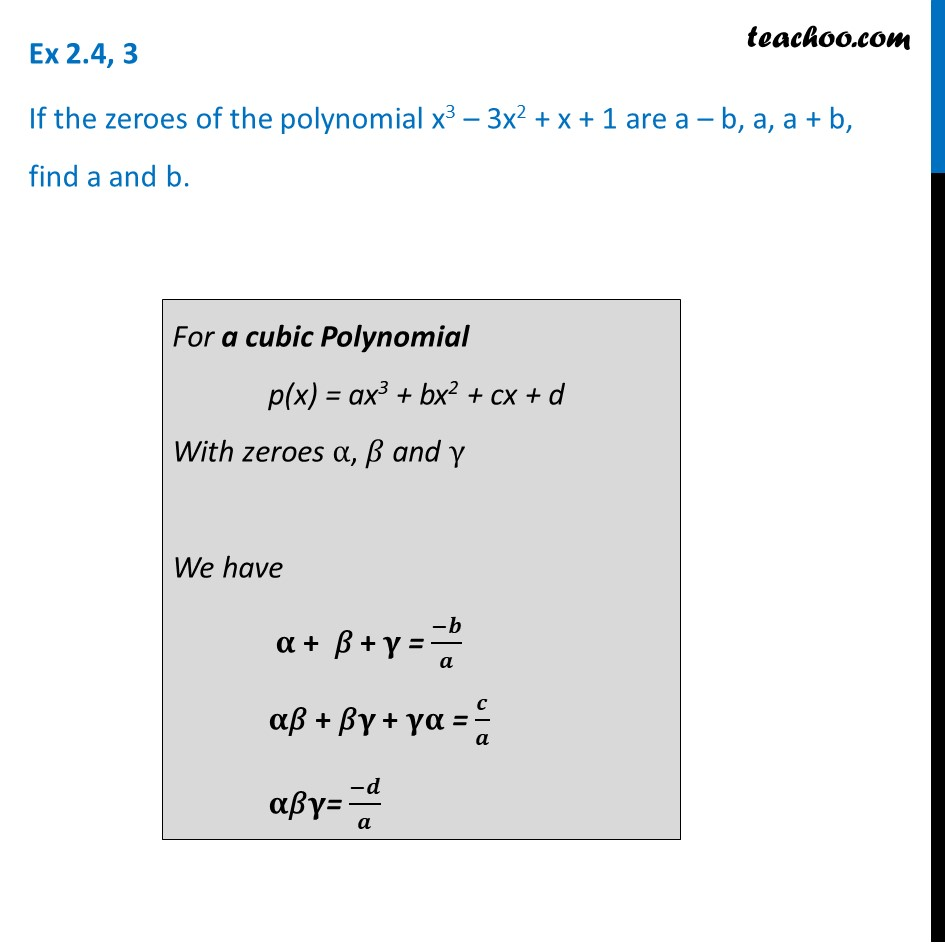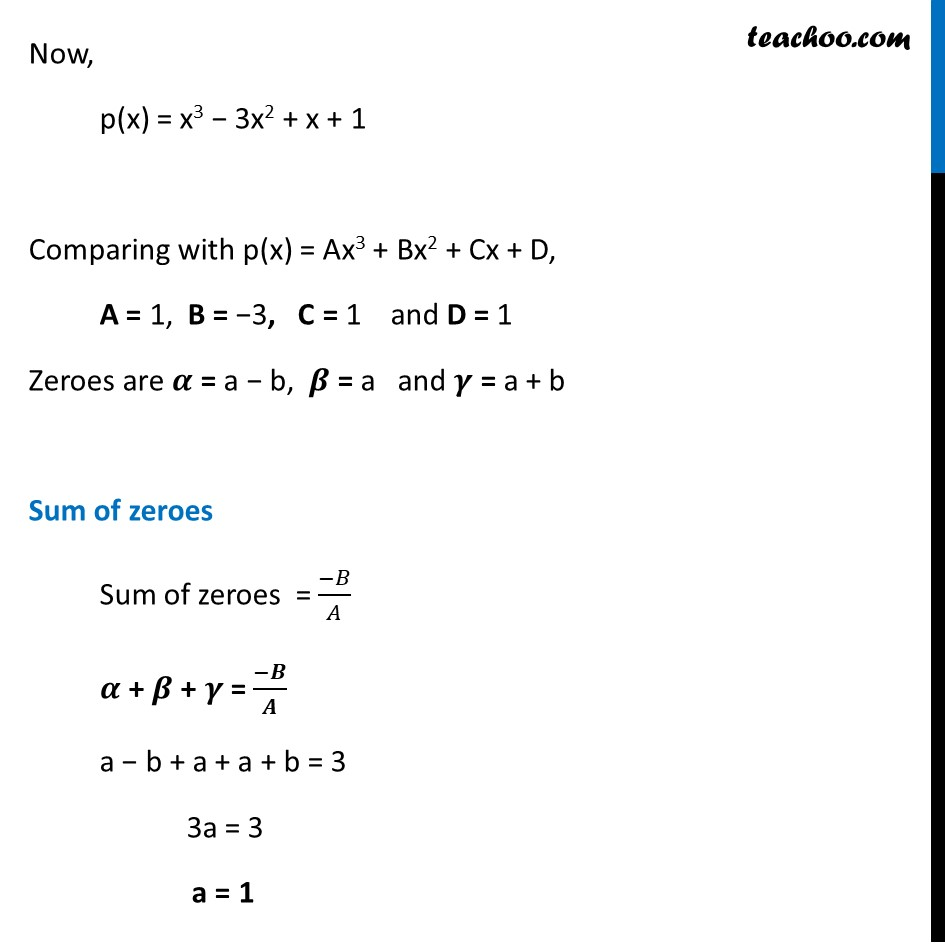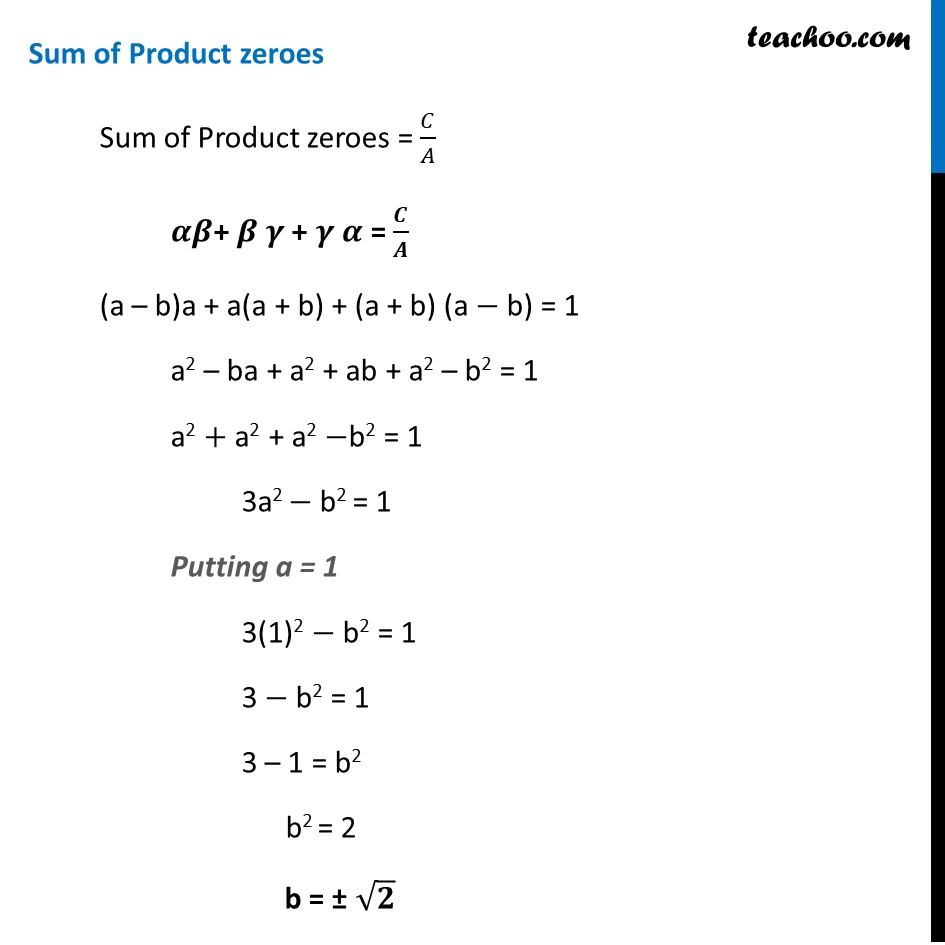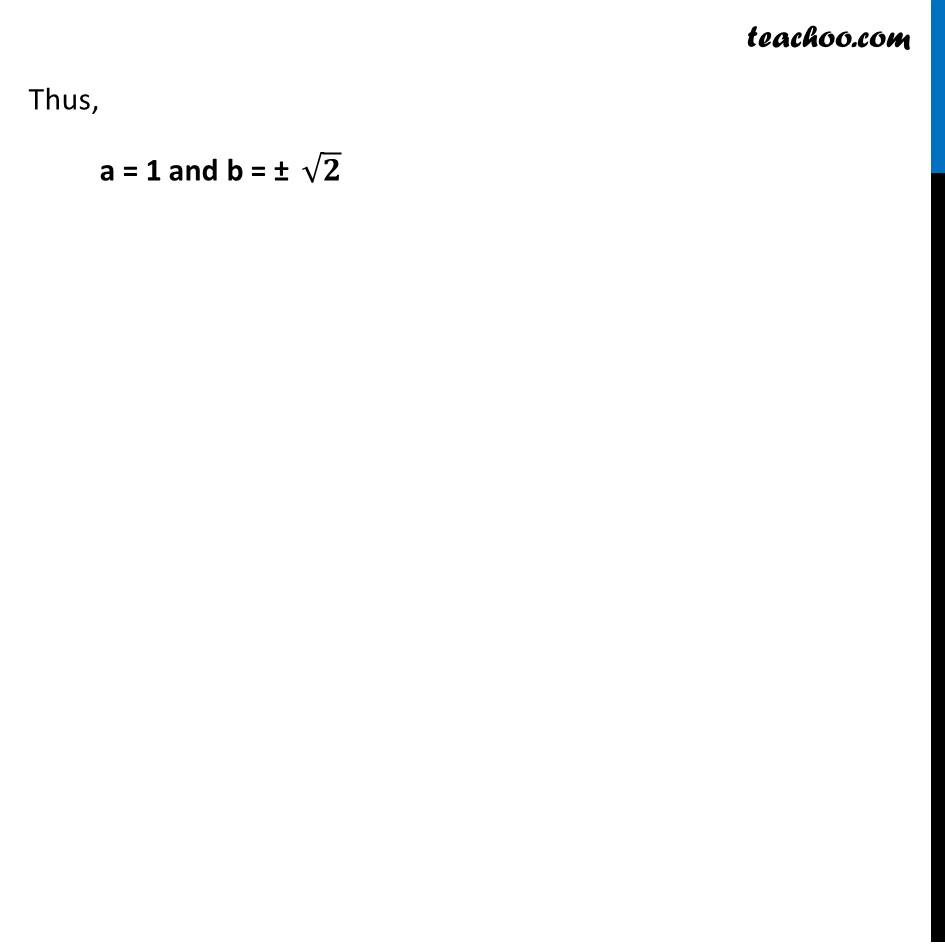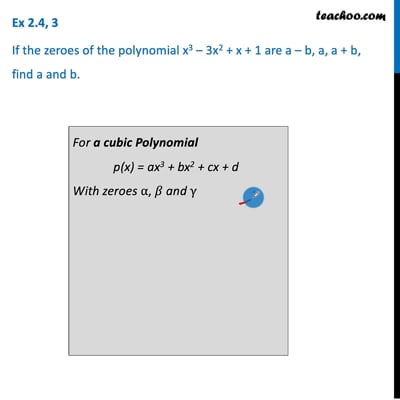This video is only available for Teachoo black users

Maths Crash Course - Live lectures + all videos + Real time Doubt solving!

### Transcript

Ex 2.4, 3 If the zeroes of the polynomial x3 – 3x2 + x + 1 are a – b, a, a + b, find a and b. For a cubic Polynomial p(x) = ax3 + bx2 + cx + d With zeroes α, 𝛽 and γ We have 𝛂 + 𝛽 + 𝛄 = (−𝒃)/𝒂 𝛂"𝛽" + 𝛽𝛄 + 𝛄𝛂 = 𝒄/𝒂 𝛂"𝛽" 𝛄= (−𝒅)/𝒂 Now, p(x) = x3 − 3x2 + x + 1 Comparing with p(x) = Ax3 + Bx2 + Cx + D, A = 1, B = −3, C = 1 and D = 1 Zeroes are 𝜶 = a − b, 𝜷 = a and 𝜸 = a + b Sum of zeroes Sum of zeroes = (−𝐵)/𝐴 𝜶 + 𝜷 + 𝜸 = (−𝑩)/𝑨 a − b + a + a + b = 3 3a = 3 a = 1 Sum of Product zeroes Sum of Product zeroes = 𝐶/𝐴 𝜶𝜷+ 𝜷 𝜸 + 𝜸 𝜶 = 𝑪/𝑨 (a – b)a + a(a + b) + (a + b) (a − b) = 1 a2 – ba + a2 + ab + a2 – b2 = 1 a2 + a2 + a2 −b2 = 1 3a2 − b2 = 1 Putting a = 1 3(1)2 − b2 = 1 3 − b2 = 1 3 – 1 = b2 b2 = 2 b = ± √𝟐 Thus, a = 1 and b = ± √𝟐